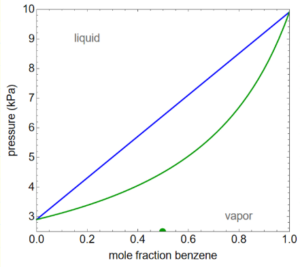LearnChemE

#### Lever Rule: Example Problems

Try to solve these problems before watching the solutions in the screencasts.

##### Example Problem 1

A liquid with a mole fraction of component 1 of 0.60 is partially evaporated in a piston-cylinder at constant pressure of 3.0 bar. At what temperature is the half the liquid evaporated? What is the composition of the liquid that remains?

##### Example Problem 2

A mixture contains 1.0 mol benzene and 1.0 mol toluene at 5.0-bar pressure at the temperature represented by the P-x-y diagram shown. How many moles of liquid are in this mixture?